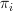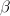Want to share your content on R-bloggers? click here if you have a blog, or here if you don't.

I recently decided to revisit some R code that implements a Gibbs sampler in an attempt to decrease the iteration time. My strategy was to implement the sampler state as an R environment rather than a list. The rationale was that passing an environment to and from functions would reduce the amount of duplication (memory copying). In my experiments, this results in only marginal improvement in iteration time. The following is just a toy example to illustrate.

Consider the logistic regression model

y_i & sim & B(pi_i) \
mathrm{logit}(pi_i) & = & beta x_i \
beta & sim & N(0, 10^{-3}),
end{array} ” />

where$y_i$ is a binary outcome, independently Bernoulli distributed with probability$pi_i$,$x_i$ is a scalar covariate, and the log odds$beta$ is a priori normal distributed with mean$0$ and precision$10^{-3}$.

For Gibbs sampling, it’s convenient to store and manupulate a model state. In the past, I had represented this in R with a list, for example

```> logit <- function(e) log(e) - log(1-e)
> expit <- function(l) exp(l) / (1+exp(l))
> # Simulate some data
> n <- 1e3
> x <- runif(n)
> y <- rbinom(n, 1, expit(5*x))
> iter <- 0
> itermax <- 1000
> state <- list(x=x, y=y, mu=0, tau=1e-3, beta=rep(0,itermax), iter=1)
```

Then, to perform a Gibbs update on beta, I would pass the old state to a function that would return the updated state. For example:

```> Gibbs <- function(state) {
+     # Metropolis update
+     state\$iter <- state\$iter + 1
+
+     old_beta  <- state\$beta[state\$iter-1]
+     old_probs <- expit(old_beta*state\$x)
+     old_logp  <- sum(log(ifelse(state\$y,old_probs,1-old_probs))) +
+                  dnorm(old_beta, state\$mu, 1/sqrt(state\$tau), log=TRUE)
+
+     new_beta  <- rnorm(1, old_beta) # Proposal
+     new_probs <- expit(new_beta*state\$x)
+     new_logp  <- sum(log(ifelse(state\$y,new_probs,1-new_probs))) +
+                  dnorm(new_beta, state\$mu, 1/sqrt(state\$tau), log=TRUE)
+
+     if(new_logp - old_logp > log(runif(1)))
+         state\$beta[state\$iter] <- new_beta
+     else
+         state\$beta[state\$iter] <- old_beta
+
+     return(state)
+ }```

Hence, the Gibbs sampler would be used as follows

```> system.time({
+ while(state\$iter < itermax)
+     state <- Gibbs(state)
+ })
user  system elapsed
2.708   0.012   2.717
> summary(state\$beta)
Min. 1st Qu.  Median    Mean 3rd Qu.    Max.
0.000   4.414   4.564   4.515   4.792   5.417```

Alternatively, the Gibbs sampler state may be stored and manipulated asan R environment. For example, to initialize the state:

```> estate <- new.env()
> assign("x", x, envir=estate)
> assign("y", y, envir=estate)
> assign("mu", 0, envir=estate)
> assign("tau", 1e-3, envir=estate)
> assign("beta", rep(0,itermax), envir=estate)
> assign("iter", 1, envir=estate)```

Then rewrite the Gibbs sampler function:

```> GibbsExp <- expression({
+     # Metropolis update
+     iter <- iter + 1
+
+     old_beta  <- beta[iter-1]
+     old_probs <- expit(old_beta*x)
+     old_logp  <- sum(log(ifelse(y,old_probs,1-old_probs))) +
+                  dnorm(old_beta, mu, 1/sqrt(tau), log=TRUE)
+
+     new_beta  <- rnorm(1, old_beta) # Proposal
+     new_probs <- expit(new_beta*x)
+     new_logp  <- sum(log(ifelse(y,new_probs,1-new_probs))) +
+                  dnorm(new_beta, mu, 1/sqrt(tau), log=TRUE)
+
+     if(new_logp - old_logp > log(runif(1)))
+         beta[iter] <- new_beta
+     else
+         beta[iter] <- old_beta
+ })
>
> eGibbs <- function(estate) {
+     eval(GibbsExp, estate)
+ }```

The Gibbs sampler is used in an almost identical manner:

```> system.time({
+ while(get("iter",envir=estate) < itermax)
+     eGibbs(estate)
+ })
user  system elapsed
2.664   0.000   2.673
> summary(get("beta",envir=estate))
Min. 1st Qu.  Median    Mean 3rd Qu.    Max.
0.000   4.378   4.588   4.556   4.779   5.704```

The percent decrease in iteration time is fairly constant for a variety of n and maxiter values. Presumably, this modest gain in speed results from less memory duplication. However, in some experiments with tracemem the story appears to be more complicated… For now, it doesn’t appear that utilizing environments is worth the trouble when the sampling algorithm is much slower than memory allocation.